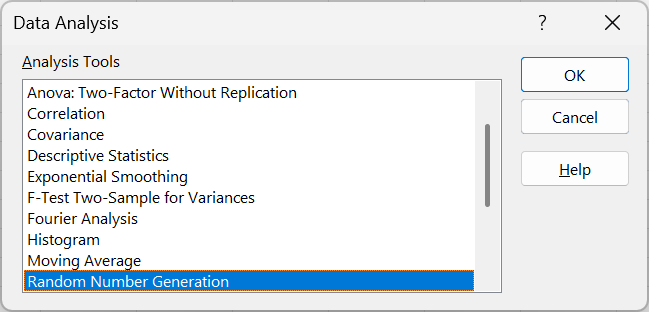# Analysis ToolPak

The Analysis-ToolPak add-in is a third party Excel add-in that provides special analysis tools suitable for medium scale statistical analysis.
This add-in should not be installed unless you are going to use the additional Data Analysis functionality as Excel will take slightly longer to open with this add-in installed.
In Excel 2003 this add-in also provided additional worksheet functions, although in Excel 2007 these were included as standard.

### Data Analysis

Once you have the Analysis-ToolPak add-in installed an extra button will appear at the on the far right of your Data tab.
This will give you a choice of the following analysis tools:Anova: Single FactorDetermines if there is a relationship between two data sets by performing an analysis of variance. Anova: Two Factor with ReplicationDetermines if there is a relationship between two data sets by performing an analysis of variance. This includes more than one sample of data for each group. Anova: Two-Factor without ReplicationDetermines if there is a relationship between two data sets by performing an analysis of variance. This does not include more than one sample of data for each group. CorrelationCompares two sets of data to determine if there is any relationship between them. CovarianceCalculates the average of the product of deviations of values from the means of each data set. Descriptive StatisticsCalculates a range of statistical measurements and summarises them into a table. This table includes calculations for: Mean, Standard Error, Median, Mode, Standard Deviation, Sample Variance, Kurtosis, Skewness, Range, Minimum, Maximum, Sum, Count, Largest, Smallest and Confidence Level. Exponential SmoothingSmooths data, weighting more recent data heavier. F-Test Two Sample for VariancesCompares the variances between two groups of data. Variance is a measure of how much the values are dispersed around the mean value. Fourier AnalysisDiscrete Fourier Transform (DFT) or Fast Fourier Transform (FFT) method, including reverse transforms. HistogramCounts occurrences in each of several data bins. Moving AverageCalculates a moving average to allow you to smooth out a data series that contains peaks. Random Number GenerationCreates a number of several types of random numbers including Uniform, Normal, Bernoulli, Poisson, Patterned and Discrete. Rank and PercentileCreates a list of the numbers ranked from highest to lowest and also provides a percentile value which indicates how an individual number ranks compared to the other numbers. RegressionCreates a table of statistics that result from least-squares regression. SamplingSamples a population randomly or periodically. T-Test: Paired Two Sample for MeansPaired two-sample students T-Test. T-Test: Two Sample assuming equal VariancesPaired two sample T-Test assuming equal means. T-Test: Two Sample assuming unequal VariancesHeteroscedastic T-Test ? Z-Test: Two Sample for MeansTwo sample Z-Test for means with known variances.

Excel 365 - C:\Program Files\Microsoft Office\Office15\Library\Analysis\PROCDB.XLAM | FUNCRES.XLAM | ANALYS32.DLL
Excel 2021 - C:\Program Files\Microsoft Office\Office15\Library\Analysis\PROCDB.XLAM | FUNCRES.XLAM | ANALYS32.DLL
Excel 2019 - C:\Program Files\Microsoft Office\Office15\Library\Analysis\PROCDB.XLAM | FUNCRES.XLAM | ANALYS32.DLL
Excel 2016 - C:\Program Files\Microsoft Office\Office15\Library\Analysis\PROCDB.XLAM | FUNCRES.XLAM | ANALYS32.DLL

### Important

There are actually two possible add-ins that can be installed relating to Analysis-ToolPak.
The add-in called Analysis-ToolPak - VBA is only needed if you are going to call the worksheet functions from VBA.